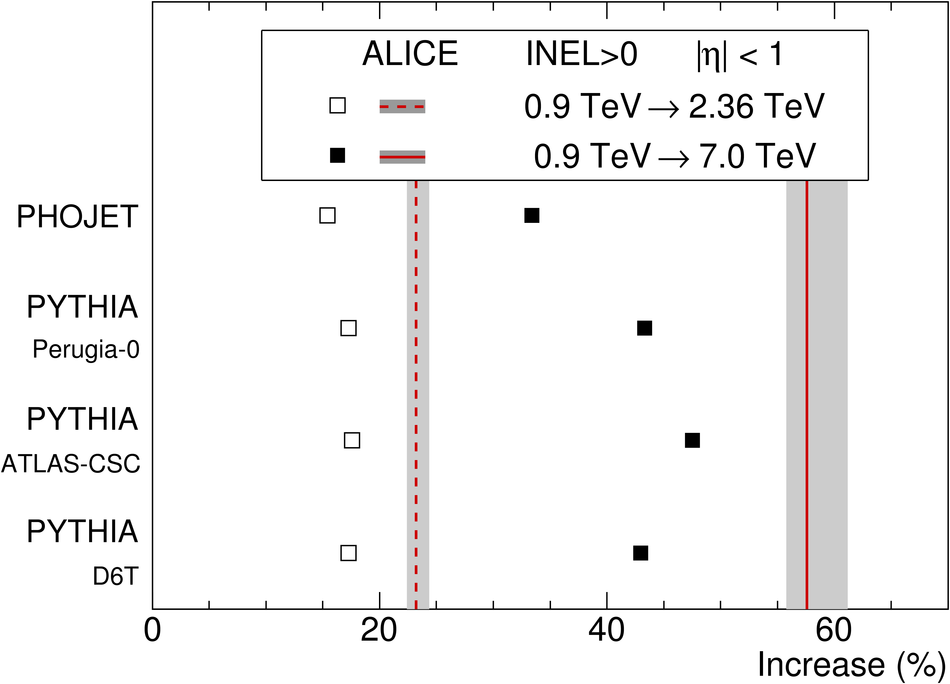# Figure 1

 Relative increase of the charged-particle pseudorapidity density, for inelastic collisions having at least one charged particle in |$\eta$| < 1, between $\sqrt{s} = 0.9~$TeV and 2.36 TeV (open squares) and between $\sqrt{s} = 0.9~$TeV and 7 TeV (full squares), for various models. Corresponding ALICE measurements are shown with vertical dashed and solid lines; the width of shaded bands correspond to the statistical and systematic uncertainties added in quadrature.